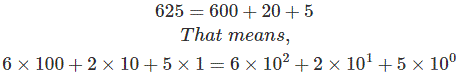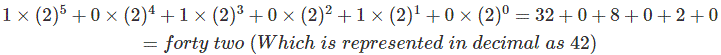# Binary Number System: What is it? (Definition & Examples)

## What is the Binary Number System?

In digital electronics Binary numbers are the most important tool for giving digital inputs. Now before understanding binary number system, we have to understand decimal number system, which we use in our daily life. The number system first introduced in human history for counting purpose.

Different numbers for counting are represented by different symbols. Such as a single thing is counted by a symbol 1, if there are two things, the symbol will be 2, for counting three things, the symbol is 3. For four, five, six, seven, eight, nine the symbols used in decimal systems are 4, 5, 6, 7, 8 and 9 respectively. Symbol 0 (zero) means there is nothing to be counted. So, 0 ,1, 2, 3, 4, 5, 6, 7, 8, 9 are ten basic symbol used to count zero to nine. That’s why it is called decimal number system or base 10 system.

But if there are more than nine things to be counted, then we do not go for another new symbol, rather we combine two or more symbols from 0 to 9 and represent the numbers which is more than nine. For representing nine + one that is ten, we use 10, for nine + two we use 11 and so on. In this way we established decimal number system.

Now coming to binary number system – the name is so because it has two base (0 and 1), which are used to represent the entire number system. See the animation below to get an idea of the conversion of binary to decimal numbers.## History of Binary Number System

The binary number system was introduced by an Indian-scholar Pingala in around 5th -2nd centuries in BC. Long and short syllables were used by him to illustrate the two types of numbers, it is more like Morse code.

Gottfried Leibniz in 1679 introduced the modern type of binary number system which we still use.
Any kind of number (decimal) can be represented by binary number system. The use of this system is popular in digital electronics because the on and off mode can be pretty easily understood by this.

00010203040506070809
10111213141516171819
20212223242526272829
30313233343536373839
40414243444546474849
50515253545556575859
60616263646566676869
70717273747576777879
80818283848586878889
90919293949596979899

Here, in the table above, every number is represented by combination of any two of basic symbols (0 to 9). These basic symbols are known as digit and corresponding position of these digit in a represented number is known as bit. So all the decimal number represented above are two digits, double bits number. The left most bit in a number is referred as most significant bit (MSB) where as the right most bit is referred as least significant bit (LSB).

If we observe the numbers given above we will find that first ten successive numbers i.e. from 00 to 09 are represented by changing ten decimal digits at LSB. Then MSB is changed to next successive digit and by changing digits at LSB other ten successive numbers (from 10 to 19) are represented. At third row in the table MSB is changed to next successive digit and by changing digits at LSB other ten successive numbers (from 20 to 29) are represented.

There are total 100 i.e. from 00 to 99 numbers represented by two bits in decimal system. 100 means 102 which implies (number of basic digits)number of bits. Hence by 3 bits 103 or 1000 numbers(i.e from 000 to 999) can be represented. Similarly, by n bits 10n numbers can be represented.

Now think about the situation, where a number system has only two digit 0 and 1. This system can also be represented in same logic as decimal system. The number system with only two digit 0 and 1 is known as binary number system.

Here, zero is represented by a symbol ‘0’ and one is represented as ‘1’ but if number is more than one then MSB is changed to 1, and by changing LSB other two successive numbers, can be represented. Just like decimal system, total numbers counted by binary system depends upon it bits.

For two bit binary, 22 numbers i.e. zero to three can be represented. Formula is same here, total numbers represented by binary number system = (number of basic digits)number of bits. Number of basic digits in binary system is two (0 and 1) but number of bits can be chosen any thing from 1 to infinity, hence all range of numbers can easily be represented by binary system, like all other number system.

## Binary Number System Definition

Binary number system is defined as a number system with a base (or radix) 2. This means that the numbers in this system are formed with two digits – 1 and 0.

Now, think about the a decimal number such as 625. From very basic concept we can understandHere, 625 is three bits decimal number, whose right most digit or digit at LSB is 5 and if we count the bits from right the right most bit will be 0. Next bit from right is numbered as 1 and here digit 2 is placed and similarly the left most bit is 2 and digit is 6. So from this example it is clear that any decimal number can be represented as:
∑digit × 10corresponding position or bit
Here in the above expression 10 is the total number of digits used in decimal system i.e. 0 to 9
Like decimal system any number system can be represented by same expression, only 10 in the expression should be replaced by total number of digits used in that system. Hence, any number in any system, can be represented as:
∑digit × (number of digits used in that system)corresponding position or bit
The number of digits used in binary number system is 2. Hence, any number can be represented in this system by:
∑digit × (2)corresponding position or bit

 5 4 3 2 1 0 → Position or bit 1 0 1 0 1 0 → Binary digits

Here, the binary number number 101010. Therefore, as per above expression, the number may be expressed as:For better understanding please observe the table below:

 Binary digits Binary Number Decimal Equivalent Position or bit 7 6 5 4 3 2 1 0 0 0 0 0 0 0 0 0 0×27 + 0×26 + 0×25 + 0×24 + 0×23 + 0×22 + 0×21 + 0×20 = 0 0 0 0 0 0 0 0 1 0×27 + 0×26 + 0×25 + 0×24 + 0×23 + 0×22 + 0×21 + 1×20 = 1 0 0 0 0 0 0 1 0 0×27 + 0×26 + 0×25 + 0×24 + 0×23 + 0×22 + 1×21 + 0×20 = 2 0 0 0 0 0 0 1 1 0×27 + 0×26 + 0×25 + 0×24 + 0×23 + 0×22 + 1×21 + 1×20 = 3 0 0 0 0 0 1 0 0 0×27 + 0×26 + 0×25 + 0×24 + 0×23 + 1×22 + 0×21 + 0×20 = 4 0 0 0 0 0 1 0 1 0×27 + 0×26 + 0×25 + 0×24 + 0×23 + 1×22 + 0×21 + 1×20 = 5 0 0 0 0 0 1 1 0 0×27 + 0×26 + 0×25 + 0×24 + 0×23 + 1×22 + 1×21 + 0×20 = 6 0 0 0 0 0 1 1 1 0×27 + 0×26 + 0×25 + 0×24 + 0×23 + 1×22 + 1×21 + 1×20 = 7 0 0 0 0 1 0 0 0 0×27 + 0×26 + 0×25 + 0×24 + 1×23 + 0×22 + 0×21 + 0×20 = 8

See the animation blow to get an idea how different binary numbers countedWant To Learn Faster? 🎓
Get electrical articles delivered to your inbox every week.
No credit card required—it’s 100% free.